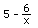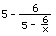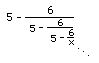2a. Get an infinite continued fraction and find approximations.
We can take this functionand in place of x we'll put, since this is equal to x and

we get. Then we'll putin for x again, and we get. If we keep doing that we get this infinite continued fraction. Jonathan, at age 7, did this at home with his Mom. He got all excited about this being infinite!
If you put 1 in the first of these you get -1. If you put 1 in the second one you get 11. If you put 1 in for x in the third one you get 4.4545.. You get an infinite sequence which approaches 3, which is one of the two roots of the equation.
If you start with -100, you would still get an infinite sequence approaching 3 as well. Notice, you only get the one root, 3, using method 2.

To order Don's materials
Back to Ch. 8, part 2- iteration
Mathman home